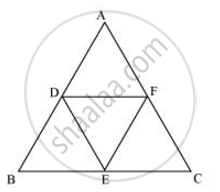# D, E and F are respectively the mid-points of sides AB, BC and CA of ΔABC. Find the ratio of the area of ΔDEF and ΔABC. - Mathematics

D, E and F are respectively the mid-points of sides AB, BC and CA of ΔABC. Find the ratio of the area of ΔDEF and ΔABC.

#### SolutionD and E are the mid-points of ΔABC

:.DE || AC and DE = 1/2AC

In ΔBED and ΔBCA

∠BED = ∠BCA   (Corresponding angle)

∠BDE = ∠BAC   (Corresponding angle)

∠EBD = ∠CBA   (Common angles)

∴ΔBED ~ ΔBCA (AAA similarity criterion)

(ar(ΔBED))/(ar(ΔBCA)) = 1/4

=>ar(ΔBED) = 1/4 ar(ΔBCA)

Similary

ar(ΔCFE) = 1/4ar(CBA)

Also ar(ΔDEF) = ar(ΔABC) -  [ar(ΔBED) + ar(ΔCFE) + ar(ΔADF)]

=>ar(ΔDEF) = ar(ΔABC) - 3/4 ar(ΔABC)  = 1/4 ar(ΔABC)

=>ar(ΔDEF) = ar(ΔABC) - 3/4 ar(ΔABC) = 1/4 ar(ΔABC)

=>(ar(ΔDEF))/(ar(ΔABC)) = 1/4

Concept: Areas of Similar Triangles
Is there an error in this question or solution?

#### APPEARS IN

NCERT Class 10 Maths
Chapter 6 Triangles
Exercise 6.4 | Q 5 | Page 144